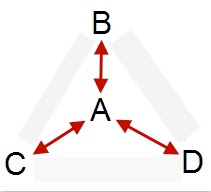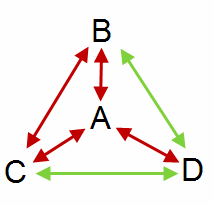## Wednesday, May 20, 2009

### Geek of the Week: Vadim Korf

Vadim wins Geek of the Week for solving one of the blog problems I wasn't able to solve. Not only solving it, but providing one of those elegant solutions that leaves you saying, "Of course, how come I couldn't think of that?" I hope you enjoy it as much as I do. Here's the problem:
A town creates a committee of six people to solve a new math problem. In the town, everyone is either a friend or an enemy. Prove that there are at least three friends or three enemies on the committee.
Six people: A, B, C, D, E, F

Two kinds of relationships: X, Y
---- r(A,B)=X means A and B have relationship of type X.
---- r(A,B,C)=X means that r(A,B)=r(A,C)=r(B,C)=X

We have to prove that we have at least one r(P1,P2,P3)=X (or Y), where P1,P2,P3 could be anyone from {A, B, C, D, E, F}

Consider A (anyone). It has a relationship of type X (could be either friend or enemy, whichever has a bigger count for A) with at least three other guys, for example B, C, D (and possibly E and/or F as well), so r(A,B)=r(A,C)=r(A,D)=X

If r(B,C)=X then r(A,B,C)=X
If r(B,D)=X then r(A,B,D)=X
If r(C,D)=X then r(A,C,D)=X
If neither 1, 2, or 3 is true, then
r(B,C)=r(B,D)=r(C,D)=Y, then r(B,C,D)=Y
If you are not picking up on his notation, basically he's saying: consider any person in the group of six. That person has a relationship with 5 other people. He/She is either friends with 3 or more people or enemies with 3 or more people. That gives you two cases. The following proof will work in either case, so we just have to look at one. Assume that A is friends with B, C, and D. Vadim has given us a diagram to help visualize the argument. The red line between any two people means they're friends.Consider the relationship between B and C, if they are friends, we have the picture. (Note: the green lines represent that the relation between them is unknown.)So A,B, and C are all friends. Therefore, there are three friends on the committee. In fact, the same is true if either BD or CD are friends. So what about if BC, CD, and BD are all enemies? Here's Vadim's picture. Remember the red lines represent friendship and the blue lines represent enmity. (Note: ignore the 4. Too lazy to edit it out.)But then B, C, and D are all enemies, so we have at least three enemies on the committee. All done.